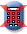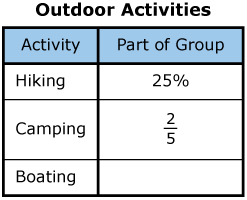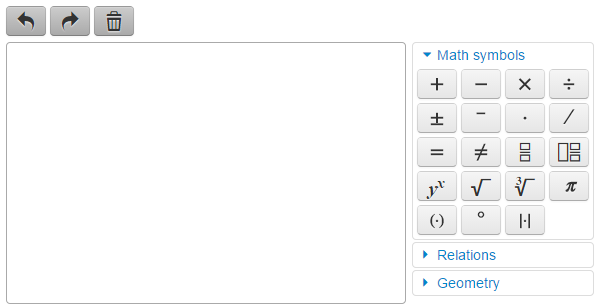Massachusetts Comprehensive Assessment SystemReporting Category: The Number System
Standard: CCSS.Math.Content.7.NS.A.3 - Solve real-world and mathematical problems involving the four operations with rational numbers. [Note: Computations with rational numbers extend the rules for manipulating fractions to complex fractions.]
Item Description: Solve a real-world problem involving the four operations on rational numbers.

Sandy leads an outdoor adventure group. She asked each member of the group to choose one activity for the next weekend. Each member chose hiking, camping, or boating. This table shows what part of the group chose hiking and what part of the group chose camping.#### Part A

What fractional part of the group chose hiking? Write your answer as a fraction in lowest terms. Show or explain how you got your answer.#### Part B

What fractional part of the group chose hiking or camping? Show or explain how you got your answer.#### Part C

What fractional part of the group chose boating? Write your answer as a fraction in lowest terms. Show or explain how you got your answer.#### Part D

There were 24 members who chose camping for the outdoor activity. What was the number of members who chose boating? Show or explain how you got your answer.## Scoring Guide and Sample Student Work

Select a score point in the table below to view the sample student response.

ScoreDescription
4 The student response demonstrates an exemplary understanding of the Number System concepts involved in solving real-world and mathematical problems involving the four operations with rational numbers. The student converts a percent to a fraction and adds, subtracts, multiplies, and divides fractions to solve a real-world problem.
4
3 The student response demonstrates a good understanding of the Number System concepts involved in solving real-world and mathematical problems involving the four operations with rational numbers. Although there is significant evidence that the student was able to recognize and apply the concepts involved, some aspect of the response is flawed. As a result the response merits 3 points.
2 The student response demonstrates a fair understanding of the Number System concepts involved in solving real-world and mathematical problems involving the four operations with rational numbers. While some aspects of the task are completed correctly, others are not. The mixed evidence provided by the student merits 2 points.
1 The student response demonstrates a minimal understanding of the Number System concepts involved in solving real-world and mathematical problems involving the four operations with rational numbers.
0 The student response contains insufficient evidence of an understanding of the Number System concepts involved in solving real-world and mathematical problems involving the four operations with rational numbers to merit any points.
Note: There are 2 sample student responses for Score Point 4.

Question 1: The Number System

Last Updated: June 22, 2020